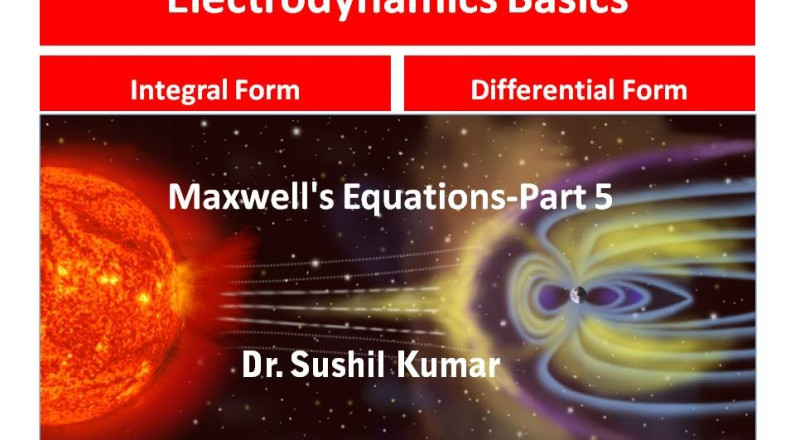In this video basic fundamentals of the Electrodynamics are discussed, Specially focused on the Maxwell’s equations, How to transform these equations from integral form to the differential form

The two basic fundamental theorems, Gauss- Divergence and Stokes theorem, are used for this purposes. In addition to this the  Gradient operator, Divergence and Curl operators are discussed,

For more information you can follow me at